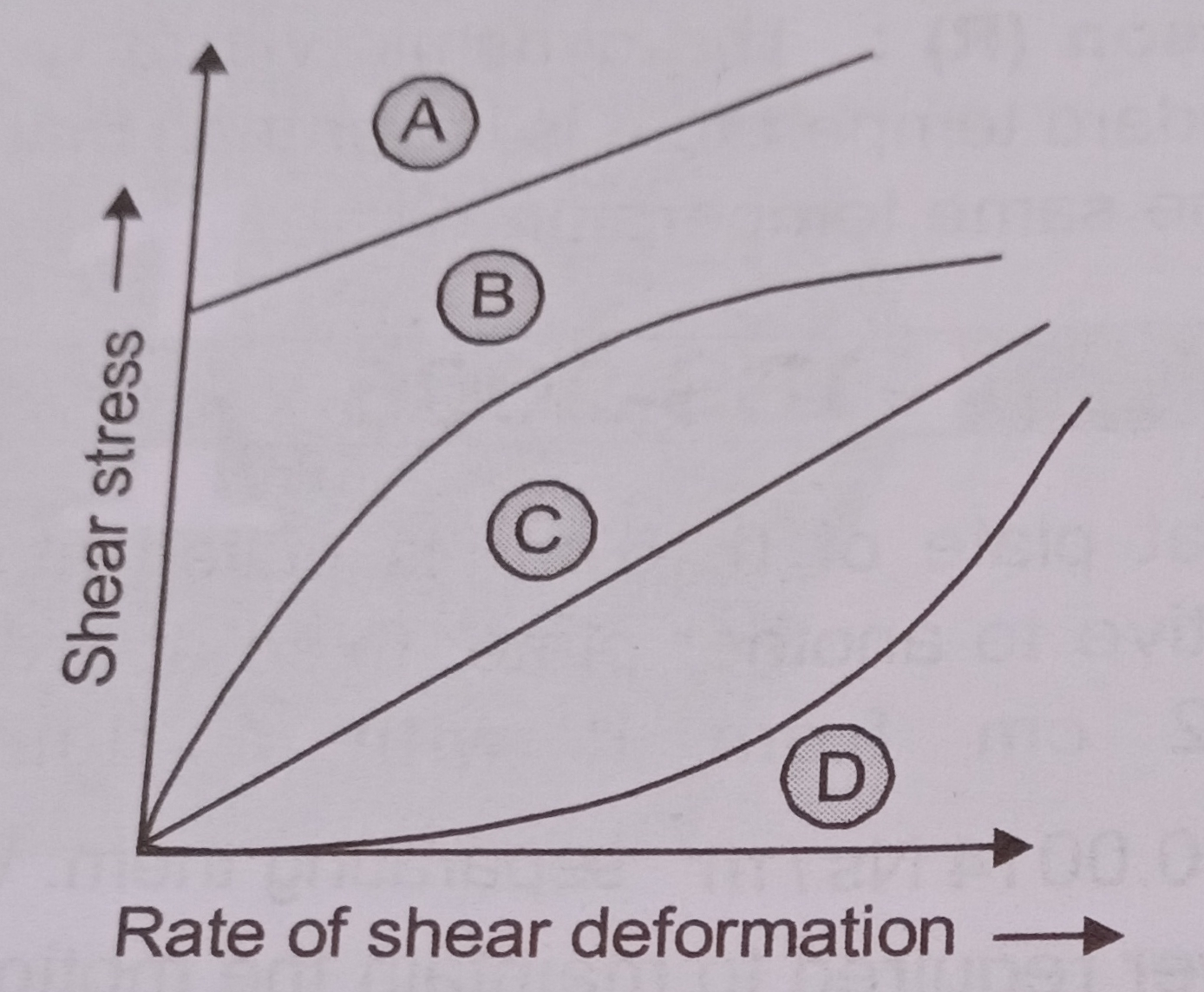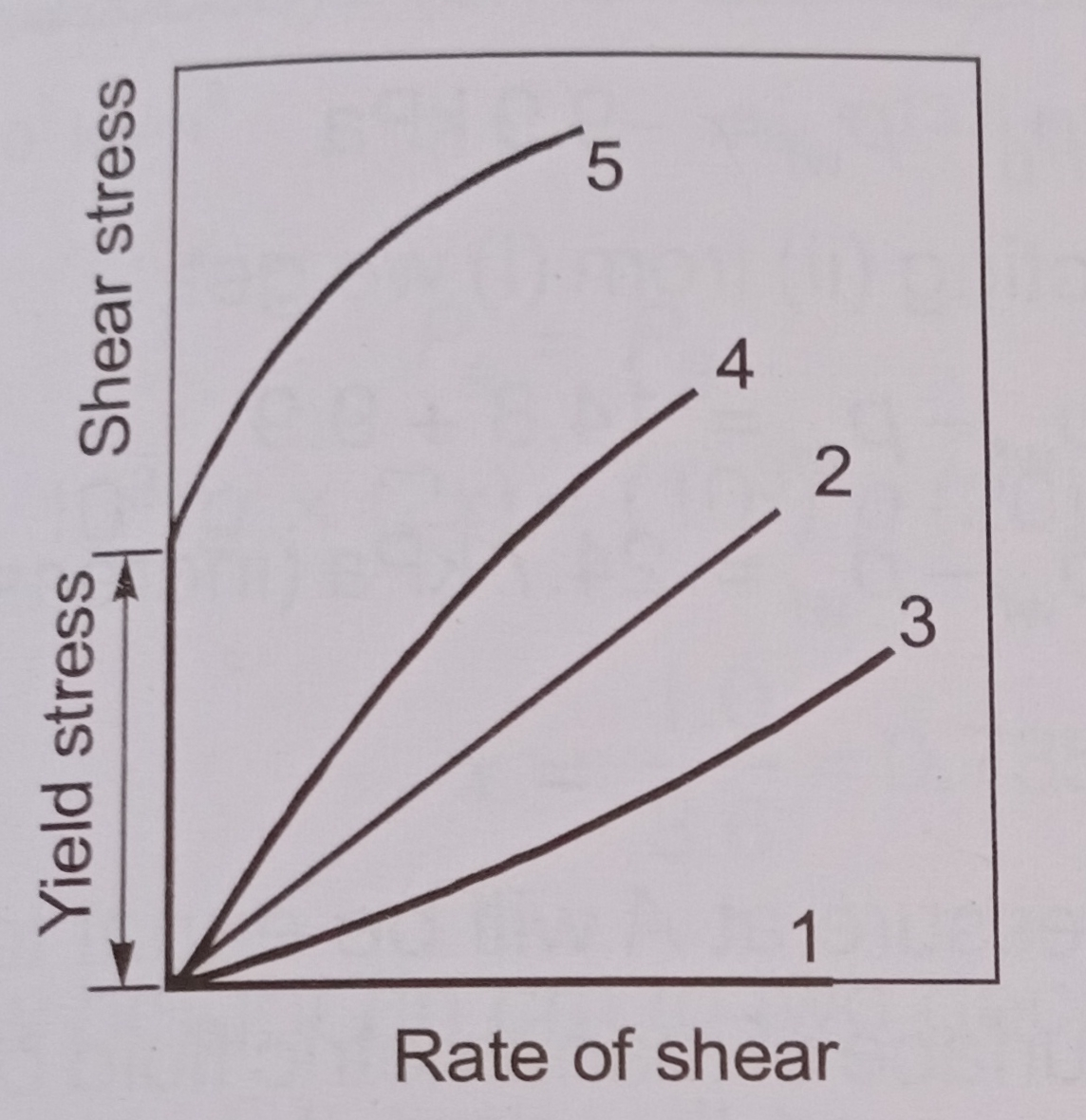# TYPES OF FLUIDS | PREVIOUS YEAR GATE & ESE MULTIPLE CHOICE QUESTIONS 9

Fluids are mainly of two types:-

1) Newtonian Fluids:

Fluids which follows Newton’s law of viscosity

2) Non-Newtonian Fluids:

These do not follow Newton’s law of viscosity.

The relation between shear stress and velocity gradient is:

where A and B are constants depending upon type of fluid and condition of flow.

The different types of fluids are as follows:

1) Dilatant Fluids:

Shear thickening fluid.

n > 1 & B = 0,

i.e. Apparent viscosity increases with increase in shear stress.

e.g.: Solution with suspended sand, conc. sugar solution, Butter, Quick sand.

2) Newtonian Fluids:

n = 1 & B = 0

i.e. Viscosity is invariant of shear stress.

e.g.: Water, air, gasoline and oil.

3) Pseudoplastic Fluids:

Shear thinning fluid

n < 1 & B = 0

i.e. Apparent viscosity decreases with increase in shear stress

e.g.: Paper pulp, Rubber solution, Lipsticks, Paints, Blood, Milk, Polymetric solutions etc.

4) Rheopectic Fluids:

n > 1 & B = 0

i.e. Apparent viscosity increases with increase in shear stress.

e.g.: Gypsum solution in water & Bentonite solution.

5) Bingham Plastic/Ideal Plactic Fluids:

n = 1 & B is not equal to  0.

e.g.: Sewage sludge, Drilling mud, tooth paste and gel.

6) For Thixotropic Fluids:

n < 1 & B is not equal to 0.

i.e., apparent viscosity decreases with

increase in shear stress.

e.g.: Printers ink and Enamels.

MULTIPLE CHOICE QUESTIONS:

Que: Match List I with List II

 List I List IIDilatant Fluid Bingham Plastic Pseudoplastic fluid Newtonian Fluid

a) A-1, B-2, C-4, D-3

b) A-1, B-2, C-3, D-4

c) A-2, B-1, C-3, D-4

d) A-2, B-1, C-4, D-3

Que: Match Group I with Group II

 Group I Group IIA. Newtonian Fluid   B. Pseudoplastic fluid   C. Plastic fluid   D. Dilatant fluid

a) 2-A, 4-B, 5-C, 3-D

b) 2-A, 1-B, 3-C, 4-D

c) 2-A, 5-B, 4-C, 1-D

d) 2-A, 4-B, 1-C, 5-D